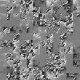author Amos RobinsonFri, 7 Dec 2012 02:16:11 +0000 (13:16 +1100) committer Amos RobinsonFri, 7 Dec 2012 02:16:11 +0000 (13:16 +1100)

index 1e09b4c..fb0f873 100644 (file)
@@ -17,6 +17,9 @@ type Char     = Word8

type String    = [: Char :]

+-- | Filter potential occurrences to find matches.
+-- For each candidate, checks @i@th character of pattern @w@ against candidate + @i@.
+-- If characters are not the same, given @candidate@ is not an occurrence of the pattern.
next_character :: [:Int:] -> String -> String -> Int -> [:Int:]
next_character candidates w s i
| i I.== lengthP w = candidates
@@ -26,6 +29,8 @@ next_character candidates w s i
(candidates',_) = unzipP (filterP (\(_,n) -> n W.== letter) (candidates `zipP` next_l))
in  next_character candidates' w s (i I.+ 1)

+-- | Find indices all occurrences of @w@ inside string @s@.
+-- > string_search "bob" "b" = [0, 2]
string_search :: String -> String -> [:Int:]
string_search w s = next_character (enumFromToP 0 (lengthP s I.- lengthP w I.+ 1)) w s 0

index 446af5e..fad86b6 100644 (file)
@@ -1,6 +1,7 @@

import Vector
--- import Vectorised
+-- Disabled: see Vectorised
+--import Vectorised
import Vector1
import Vectorised1
import Timing
@@ -32,9 +33,11 @@ run  :: String
-> Int                         -- ^ How many points to use.
-> IO ()

-{-
+
run "vectorised" pointCount
= do
+   putStrLn "Disabled: see Vectorised.hs"
+ {-
vPoints <- pointsPArrayOfUArray
\$ genPointsDisc pointCount (400, 400) 350

@@ -49,7 +52,8 @@ run "vectorised" pointCount

-- Print how long it took.
putStr \$ prettyTime tElapsed
--}
+    -}
+

run "vector" pointCount
= do
index 65feb5b..59846c3 100644 (file)
@@ -26,6 +26,9 @@ closeststupid pts
m2 a b c = distancex a b `compare` distancex a c

+-- | Find the points within distance @d@ of the edge along x=@x0@.
+-- Only the first element of each pair is checked.
+-- Returns pairs with indices within original input array.
near_boundary :: U.Vector (Point,Point) -> Double -> Double -> U.Vector (Int,(Point,Point))
near_boundary a x0 d
= U.filter check (U.indexed a)
@@ -33,6 +36,8 @@ near_boundary a x0 d
check (_,((x1,_),_)) = abs (x1 - x0) < d

+-- | Given two arrays of pairs where the firsts are both near some boundary,
+-- update the first array with any closer points in the second array.
new_nearest :: U.Vector (Int,(Point,Point)) -> U.Vector (Int,(Point,Point)) -> U.Vector (Int,(Point,Point))
new_nearest a b
| U.length b == 0 = a
@@ -48,6 +53,11 @@ new_nearest a b
then pn
else pt2

+-- | Merge two arrays of pairs that have been split on the x axis.
+-- To do this, we find the maximum distance between any two points,
+-- then find the points in each array within that distance of the split.
+-- We check each of these boundary points against the other boundary
+-- to see if they are closer.
merge_pairs :: Double -> U.Vector (Point,Point) -> U.Vector (Point,Point) -> U.Vector (Point,Point)
merge_pairs x0 a b
= let d  = sqrt (max (U.maximum (U.map dist a)) (U.maximum (U.map dist b)))
@@ -60,6 +70,11 @@ merge_pairs x0 a b
dist (a,b) = distancex a b

+-- | For each point, find its closest neighbour.
+-- Once there are few enough points, we use a naive n^2 algorithm.
+-- Otherwise, the median x is found and the array is split into
+-- those below and those above.
+-- The two halves are recursed upon and then the results merged (see @merge_pairs@).
closest :: U.Vector Point -> U.Vector (Point,Point)
closest pts
| U.length pts < 250 = closeststupid pts
@@ -90,9 +105,17 @@ closeststupidV :: U.Vector Point -> U.Vector (Point,Point)
closeststupidV = closeststupid

+-- | Find median of an array, using quickselect
median :: U.Vector Double -> Double
median xs = median' xs (U.length xs `div` 2)

+-- | Find the @k@th smallest element.
+-- A pivot is selected and the array is partitioned into those smaller and larger than the pivot.
+-- If the number of elements smaller than pivot is greater or equal to @k@, then the pivot must be larger than the
+-- @k@th smallest element, and we recurse into the smaller elements.
+-- Otherwise the @k@th element must be larger or equal to the pivot.
+-- Since lesser elements are not in the greater array, we are no longer looking for the
+-- @k@th smallest element, but the @k - (length xs - length gs)@th smallest.
median':: U.Vector Double -> Int -> Double
median' xs k =
let p  = xs U.! (U.length xs `div` 2)
index 5a94f01..7e8d470 100644 (file)
@@ -1,3 +1,6 @@
+-- Vectorised "all closest pairs":
+-- This was
+
{-# LANGUAGE ParallelArrays #-}
{-# OPTIONS -fvectorise #-}
{-# OPTIONS -fno-spec-constr-count #-}
@@ -8,11 +11,39 @@ import Data.Array.Parallel.Prelude.Double        as D
import qualified Data.Array.Parallel.Prelude.Int as I
import qualified Prelude as P

+--------- dph-lifted-copy implementations
+-- These functions are in -copy but not in -vseg.
+-- I've tried to implement them but got a vectorisation error:
+--  "Can't vectorise expression GHC.Prim.Int#"
+--
+bpermuteP :: [:a:] -> [:Int:] -> [:a:]
+bpermuteP as is = mapP (\i -> as !: i) is
+
+indexedP :: [:a:] -> [:(Int,a):]
+indexedP xs = zipP (I.enumFromToP 0 (lengthP xs I.- 1)) xs
+
+updateP :: [:a:] -> [:(Int,a):] -> [:a:]
+updateP as is = snd' (unzipP (mapP find (indexedP as)))
+ where
+  find (i,a)
+   | [:v:] <- filterP (\(i',_) -> i I.== i') is
+   = v
+   | otherwise
+   = (i,a)
+
+  snd' (_,b) = b
+
+
+
+-- | Distance squared.
+-- Because most uses of this are just finding the minimum, the sqrt is unnecessary.
distance :: Point -> Point -> Double
distance (x1, y1) (x2, y2)
=   (x2 D.- x1) D.* (x2 D.- x1)
D.+ (y2 D.- y1) D.* (y2 D.- y1)

+
+-- | Distance squared.
-- Distance between two points, but return a very large number if they're the same...
distancex :: Point -> Point -> Double
distancex a b
@@ -20,8 +51,7 @@ distancex a b
in  if d D.== 0 then 1e100 else d

-bpermuteP as is = mapP (\i -> as !: i) is
-
+-- | Naive closest pairs.
-- An n^2 algorithm for finding closest pairs.
-- Our divide and conquer drops back to this once there are few enough points
closeststupid :: [: Point :] -> [:(Point,Point):]
@@ -29,14 +59,27 @@ closeststupid pts
= let is = [: minIndexP [: distancex a b | b <- pts :] | a <- pts :]
in  bpermuteP [: (a,b) | a <- pts, b <- pts :] is

-near_boundary :: [:(Point,Point):] -> Double -> Double -> [:(Int,(Point,Point)):]
+
+-- | Find the points within distance @d@ of the edge along x=@x0@.
+-- Only the first element of each pair is checked.
+-- Returns pairs with indices within original input array.
+near_boundary
+    :: [:(Point,Point):]        -- ^ Input pairs
+    -> Double                   -- ^ X dimension of boundary
+    -> Double                   -- ^ Maximum distance from X boundary
+    -> [:(Int,(Point,Point)):]
near_boundary a x0 d
= filterP check (indexedP a)
where
-  check (_,((x1,_),_)) = abs (x1 - x0) < d
+  check (_,((x1,_),_)) = D.abs (x1 D.- x0) D.< d

-new_nearest :: [:(Int,(Point,Point)):] -> [:(Int,(Point,Point)):] -> [:(Int,(Point,Point)):]
+-- | Given two arrays of pairs where the firsts are both near some boundary,
+-- update the first array with any closer points in the second array.
+new_nearest
+    :: [:(Int,(Point,Point)):] -- ^ Input pairs
+    -> [:(Int,(Point,Point)):] -- ^ Check each input pair against these to find any closer pairs
+    -> [:(Int,(Point,Point)):]
new_nearest a b
| lengthP b I.== 0 = a
| otherwise
@@ -46,13 +89,22 @@ new_nearest a b
in  na
where
check pt1 pn pt2
-   = if   distance pt1 pn < distance pt1 pt2
+   = if   distance pt1 pn D.< distance pt1 pt2
then pn
else pt2

-merge_pairs :: Double -> [:(Point,Point):] -> [:(Point,Point):] -> [:(Point,Point):]
+-- | Merge two arrays of pairs that have been split on the x axis.
+-- To do this, we find the maximum distance between any two points,
+-- then find the points in each array within that distance of the split.
+-- We check each of these boundary points against the other boundary
+-- to see if they are closer.
+merge_pairs
+    :: Double            -- ^ Split point
+    -> [:(Point,Point):] -- ^ `Above' pairs
+    -> [:(Point,Point):] -- ^ `Below' pairs
+    -> [:(Point,Point):]
merge_pairs x0 a b
- = let d  = sqrt (max (maximumP (mapP dist a)) (maximumP (mapP dist b)))
+ = let d  = sqrt (D.max (maximumP (mapP dist a)) (maximumP (mapP dist b)))
an = near_boundary a x0 d
bn = near_boundary b x0 d
a' = a `updateP` new_nearest an bn
@@ -62,6 +114,11 @@ merge_pairs x0 a b
dist (a,b) = distance a b

+-- | For each point, find its closest neighbour.
+-- Once there are few enough points, we use a naive n^2 algorithm.
+-- Otherwise, the median x is found and the array is split into
+-- those below and those above.
+-- The two halves are recursed upon and then the results merged (see @merge_pairs@).
closest :: [:Point:] -> [:(Point,Point):]
closest pts
| lengthP pts I.< 250 = closeststupid pts
@@ -71,8 +128,6 @@ closest pts
xd   = maximumP xs D.- minimumP xs
yd   = maximumP ys D.- minimumP ys

-       pts' = if yd D.> xd then flip pts else pts
-
mid  = median xs

top  = filterP (\(x,_) -> x D.>= mid) pts
@@ -82,17 +137,7 @@ closest pts
bot' = closest bot

pair = merge_pairs mid top' bot'
-   in  if yd D.> xd then flip2 pair else pair
-
-flip pts
- = let (xs,ys) = unzipP pts
-   in  zipP xs ys
-
-flip2 prs
- = let (p1,p2) = unzipP prs
-       (x1,y1) = unzipP p1
-       (x2,y2) = unzipP p2
-   in  (zipP y1 x1) `zipP` (zipP y2 x2)
+   in  pair

closestPA :: PArray Point -> PArray (Point,Point)
@@ -102,9 +147,17 @@ closeststupidPA :: PArray Point -> PArray (Point,Point)
closeststupidPA ps = toPArrayP (closeststupid (fromPArrayP ps))

+-- | Find median of an array, using quickselect
median :: [: Double :] -> Double
median xs = median' xs (lengthP xs `I.div` 2)

+-- | Find the @k@th smallest element.
+-- A pivot is selected and the array is partitioned into those smaller and larger than the pivot.
+-- If the number of elements smaller than pivot is greater or equal to @k@, then the pivot must be larger than the
+-- @k@th smallest element, and we recurse into the smaller elements.
+-- Otherwise the @k@th element must be larger or equal to the pivot.
+-- Since lesser elements are not in the greater array, we are no longer looking for the
+-- @k@th smallest element, but the @k - (length xs - length gs)@th smallest.
median':: [: Double :] -> Int -> Double
median' xs k =
let p  = xs !: (lengthP xs `I.div` 2)
index d8336ee..cd08897 100644 (file)
@@ -28,19 +28,38 @@ closeststupid pts
= let i = minIndexP [: distancex a b | a <- pts, b <- pts :]
in  [: (a,b) | a <- pts, b <- pts :] !: i

-near_boundary :: [:Point:] -> Double -> Double -> [:Point:]
+
+-- | Find the points within distance @d@ of the edge along x=@x0@.
+near_boundary
+    :: [:Point:]    -- ^ array of points
+    -> Double       -- ^ split / x boundary
+    -> Double       -- ^ maximum distance
+    -> [:Point:]
near_boundary pts x0 d
= filterP check pts
where
check (x1,_) = D.abs (x1 D.- x0) D.< d

+-- | Find pair with minimum distance between tops * bots
merge :: [:Point:] -> [:Point:] -> (Point,Point)
merge tops bots
= let i = minIndexP [: distancex a b | a <- tops, b <- bots :]
in  [: (a,b) | a <- tops, b <- bots :] !: i

-merge_pairs :: Double -> [:Point:] -> [:Point:] -> (Point,Point) -> (Point,Point) -> (Point,Point)
+
+-- | Given closest pairs in points above and below split,
+-- we want to find the minimum of all points.
+-- To do this, we find the points within a certain distance
+-- from the split boundary, and check them against each other.
+-- We then take the minimum of all points.
+merge_pairs
+    :: Double           -- ^ split / x boundary
+    -> [:Point:]        -- ^ points above split
+    -> [:Point:]        -- ^ points below split
+    -> (Point,Point)    -- ^ closest pair in points above
+    -> (Point,Point)    -- ^ closest pair in points below
+    -> (Point,Point)
merge_pairs x0 top bot (a1,a2) (b1,b2)
= let da   = distancex a1 a2
db   = distancex b1 b2
@@ -59,6 +78,10 @@ merge_pairs x0 top bot (a1,a2) (b1,b2)
in if dm D.< mind then (m,n) else min2

+-- | Find closest two points in array of points.
+-- Use naive n^2 algorithm when there are few points,
+-- otherwise split along median X and do each half recursively.
+-- And merge the result of the two halves.
closest :: [:Point:] -> (Point,Point)
closest pts
| lengthP pts I.< 250 = closeststupid pts
@@ -68,25 +91,17 @@ closest pts
xd   = maximumP xs D.- minimumP xs
yd   = maximumP ys D.- minimumP ys

-       pts' = pts -- if yd > xd then flip pts else pts
-
mid  = median xs

-       top  = filterP (\(x,_) -> x D.>= mid) pts'
+       top  = filterP (\(x,_) -> x D.>= mid) pts
-- NOTE was error here "combine2SPack" when "not (x >= mid)"
-       bot  = filterP (\(x,_) -> x D.< mid) pts'
+       bot  = filterP (\(x,_) -> x D.< mid) pts

top' = closest top
bot' = closest bot

pair = merge_pairs mid top bot top' bot'
-   in  pair -- if yd > xd then flip2 pair else pair
-
-flip pts
- = let (xs,ys) = unzipP pts
-   in  zipP xs ys
-
-flip2 ((x1,y1),(x2,y2)) = ((y1,x1),(y2,x2))
+   in  pair

closest1PA :: PArray Point -> (Point,Point)
index 3033bc9..f06087d 100644 (file)
@@ -24,6 +24,8 @@ solvePA
solvePA vertices triangles rays time
= toPArrayP (solveV (fromPArrayP vertices) (fromPArrayP triangles) (fromPArrayP rays) time)

+
+-- | Cast all rays into triangle mesh
solveV
:: [:Vec3:]             -- ^ vertices of the surface
-> [:(Int,Int,Int):]    -- ^ triangles, each 3 vertex indices
@@ -36,6 +38,7 @@ solveV vertices triangles rays time
cast' = cast vertices triangles time

+-- | Cast a single ray into the rotated triangle mesh
cast
:: [:Vec3:]          -- ^ vertices of the surface
-> [:(Int,Int,Int):] -- ^ triangles, each 3 vertex indices
@@ -49,10 +52,13 @@ cast vertices triangles time ray
(mapP (\t -> check r' pl (tri vertices t time)) triangles)
in  (ray, mi)

+-- | Return scale / distance along line's direction for intersection. Or a large number if there is no intersection.
+check :: Line -> Pluecker -> Triangle -> Double
check r pl t
| inside pl t = lineOnTriangle r t
| otherwise = 1e100

+-- Get all triangle points from mesh, rotated depending on time
tri :: [:Vec3:] -> (Int,Int,Int) -> Double -> Triangle
tri v (a,b,c) time = (get a, get b, get c)
where
index c15fdb3..d08103e 100644 (file)
@@ -13,6 +13,13 @@ quickselectPA:: PArray Double -> Int -> Double
quickselectPA xs k = qselectVect' (fromPArrayP xs) k

+-- | Find the @k@th smallest element.
+-- A pivot is selected and the array is partitioned into those smaller and larger than the pivot.
+-- If the number of elements smaller than pivot is greater or equal to @k@, then the pivot must be larger than the
+-- @k@th smallest element, and we recurse into the smaller elements.
+-- Otherwise the @k@th element must be larger or equal to the pivot.
+-- Since lesser elements are not in the greater array, we are no longer looking for the
+-- @k@th smallest element, but the @k - (length xs - length gs)@th smallest.
qselectVect':: [: Double :] -> Int -> Double
qselectVect' xs k =
let p  = xs !: (lengthP xs `I.div` 2)
index a7a8535..e306d3c 100644 (file)
@@ -14,11 +14,10 @@ import Data.Array.Parallel.Prelude.Double        as D hiding (pi)
import qualified Data.Array.Parallel.Prelude.Int as I
import qualified Prelude    as P

-{-
-data NodeV = NodeV Double Double Double [:NodeV:]
--}

+-- pi: work around strange vectoriser error
pi = 3.1415926535
+
type Point = (Double,Double)

{-# NOINLINE solvePA #-}
@@ -30,7 +29,13 @@ solvePA depth t
= let s p   = solveV2 t depth p
in toPArrayP (s 0 +:+ s (pi/2) +:+ s pi +:+ s (3*pi / 2))

-solveV2 :: Double -> Int -> Double -> [:Point:]
+
+-- | Draw a simple, rotated tree.
+solveV2
+    :: Double       -- ^ current time / rotation
+    -> Int          -- ^ number of children
+    -> Double       -- ^ phase offset
+    -> [:Point:]
solveV2 t iG pG
= let
{-# INLINE l #-}
@@ -50,30 +55,8 @@ solveV2 t iG pG
{-# INLINE pts #-}
pts       = concatP (mapP (\iG2 -> solveV2 t (iG I.- 1) (fromInt iG2 / l * 2 * pi - pi)) (I.enumFromToP 1 iG))
{-# INLINE pts' #-}
+       -- Rotate children
pts'      = mapP (\(x,y) -> (x * cos' - y * sin' + px, x * sin' + y * cos' + py)) pts
in singletonP (px, py) +:+ pts'

-{-
-mkNode :: Int -> Double -> NodeV
-mkNode i p
- = let i' = fromInt i
-   in  NodeV i' p (i' / 20)
-       (mapP (\i2 -> mkNode (i I.- 1) (fromInt i2 / i' * 2 * pi - pi)) (I.enumFromToP 1 i))
-
-
-{-# INLINE solveV #-}
-solveV :: Double -> NodeV -> [:Point:]
-solveV t (NodeV l p f c)
- = let r'        = p + f*t
-       cos'      = cos r'
-       sin'      = sin r'
-       rot (x,y) = (x * cos' - y * sin', x * sin' + y * cos')
-       (px, py)  = rot (0, l)
-       trn (x,y) = (x + px, y + py)
-
-       pts       = concatP (mapP (solveV t) c)
-       pts'      = mapP (\p -> trn (rot p)) pts
-   in singletonP (px, py) +:+ pts'
-
--}